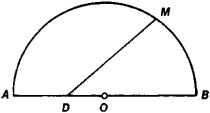# Kepler's Equation

The following article is from The Great Soviet Encyclopedia (1979). It might be outdated or ideologically biased.

## Kepler’s Equation

a transcendental equation of the form

yc sin y = x

The case |c| < 1 is important for applications, when is y determined from c and x in a unique manner. The equation was first examined by J. Kepler (Astronomía nova, 1609) in connection with the following problem: point D is given on the diameter AB of the semicircle AOBM; draw a line DM such that it divides theFigure 1

area of the semicircle in a given ratio (see Figure 1). Kepler’s equation plays an important role in astronomy in the determination of the elements of the elliptical orbits of the planets. In celestial mechanics the equation is usually written in the form

E – e sin E = M

where e is the eccentricity of the ellipse, M is the mean anomaly, and E is the eccentric anomaly. The solution of Kepler’s equation also occupied J. Lagrange (1771), P. Laplace (1823), F. Bessel (1816–17), K. Gauss (1809), and others.

### REFERENCE

Subbotin, M. F. Kurs nebesnoi mekhaniki, 2nd ed., vol. 1. Leningrad-Moscow, 1941.